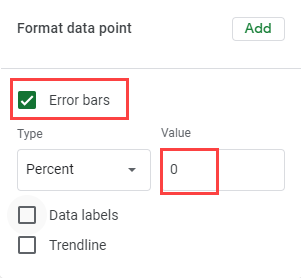# Calculate Cumulative Percentage – Excel and Google Sheets

This tutorial will demonstrate how to calculate cumulative percentage in Excel and Google Sheets.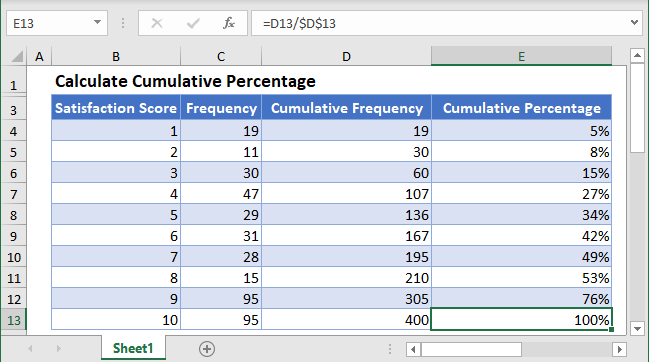A Frequency Distribution is a distribution of the number of occurrences of a set of events. In other words, a frequency distribution shows different values in a dataset and the number of times the values occur in the dataset.A Cumulative Frequency Distribution shows the total number of occurrences of the events not higher (or not lower) than a particular event in a given set of events. In other words, a Cumulative Frequency Distribution shows the number of observations that lie from a value and above (or below) the value in a dataset.

The Cumulative Percentage shows the percentage of the number of occurrences of an event and the events below it compared to the total number of all events. In other words, a Cumulative Frequency Distribution shows the percentage of the number of observations that lie not higher (or not lower) than a value in a dataset.

The Cumulative Percentage is calculated by dividing the cumulative frequency by the total frequency. That is, by dividing the sum of the frequency of an event and the frequencies before it (or after it) by the total frequency. The cumulative percentage of the last class or event must be equal to .

## Cumulative Percentage Formula

The cumulative percentage is calculated using the formula: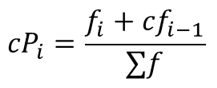where  fi+cfi-1  is the cumulative frequency of each event, value, or class;

fi is the number of occurrence (frequency) of the event, value, or class;

cfi-1  is the cumulative frequency of the preceding event, value, or class; and

Σf  is the total frequency.

## How to Calculate Cumulative Percentage in Excel

Background: An Internet Service Provider is conducting a customer satisfaction survey from a random sample of its users. They asked each surveyed customer to rate their services in the past 90 days on a scale of 1 to 10, where 10 represents excellent, and 1 represents miserable. The results from the survey are collated and presented in the table below. Calculate the cumulative percentage of the result of the survey.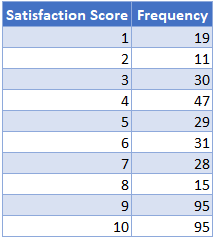First, calculate the cumulative frequency distribution of the scores by adding each frequency to the preceding frequencies.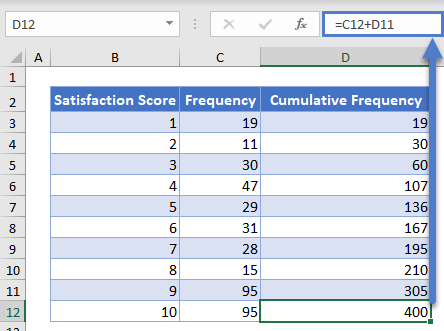Note: The last value of the Cumulative Frequency column must be equal to the sum of all frequencies. That is, the last value of the Cumulative Frequency Distribution is equivalent to the total frequency.

Next, calculate the cumulative percentage by dividing each cumulative frequency by the total frequency (the last value of the Cumulative Frequency column).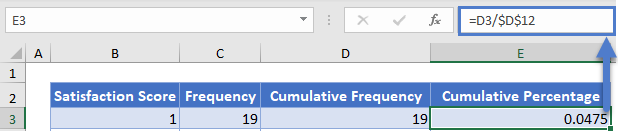Note: Double-Click the bottom right corner of the cell to fill down the data to the rest of the column.

The complete Cumulative Percentage column is shown below.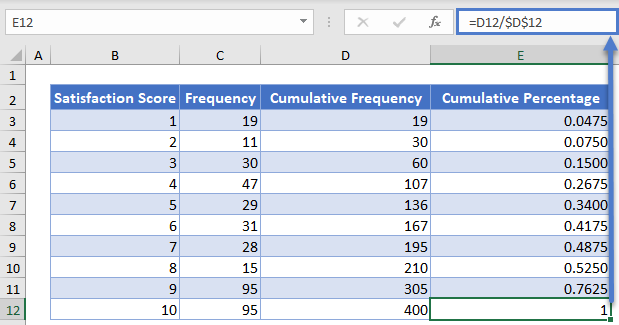Next, convert the Cumulative Percentages to percentages by selecting the Cumulative Percentage column and clicking the ‘Percent Style’ button or clicking CTRL+SHIFT+%.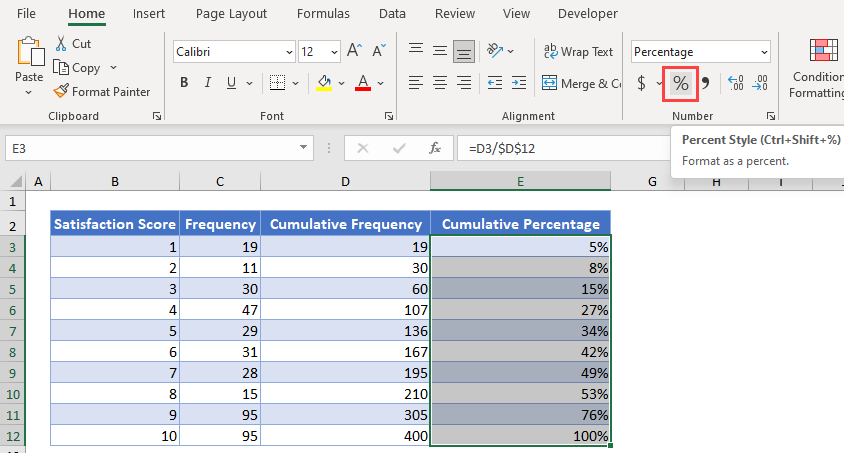The Cumulative Percentage graph looks exactly like the Cumulative Frequency Distribution graph except for the change in the vertical axis labels.

To visualize the cumulative percentage graph, you can create a cumulative percentage curve (ogive) following the steps shown below.

Hold down the CTRL key and select the Satisfaction Score and the Cumulative Percentage columns. Then, click on the Scatter with Smooth Lines and Markers chart in the Insert Scatter (X, Y) or Bubble Chart chart type on the Charts group of the Insert tab.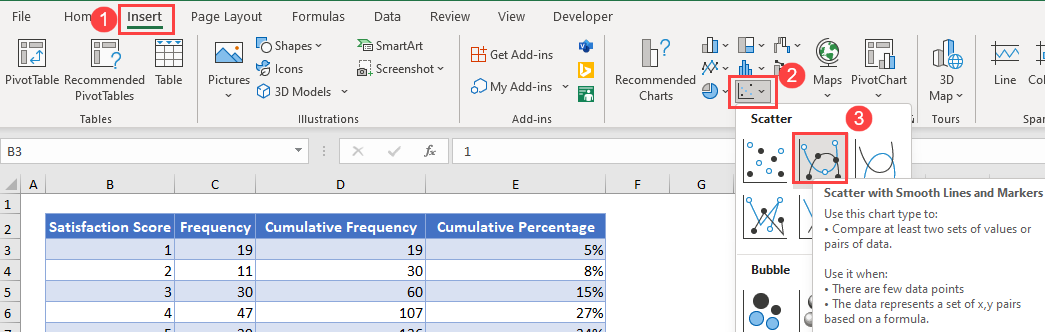The resulting scatter (ogive) chart is shown below.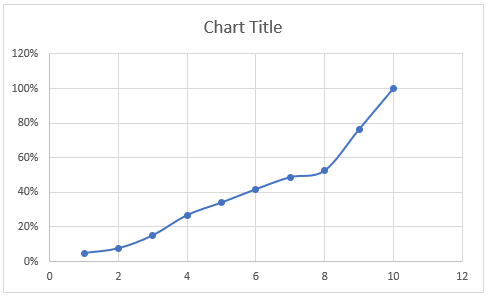Edit the chart title by clicking on the Chart Title in the chart and typing your desired title.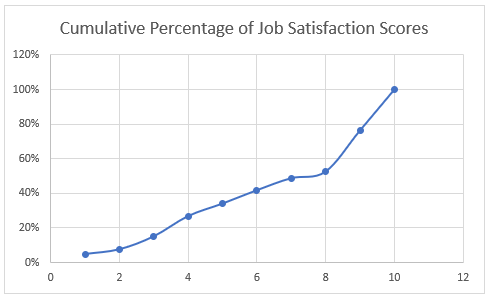Add the horizontal and the vertical axis titles by selecting the chart, then click on the Chart Design tab, then on the Add Chart Element button, then on Axis Title, and then on Primary Horizontal for the horizontal axis title or on Primary Vertical for the vertical axis title.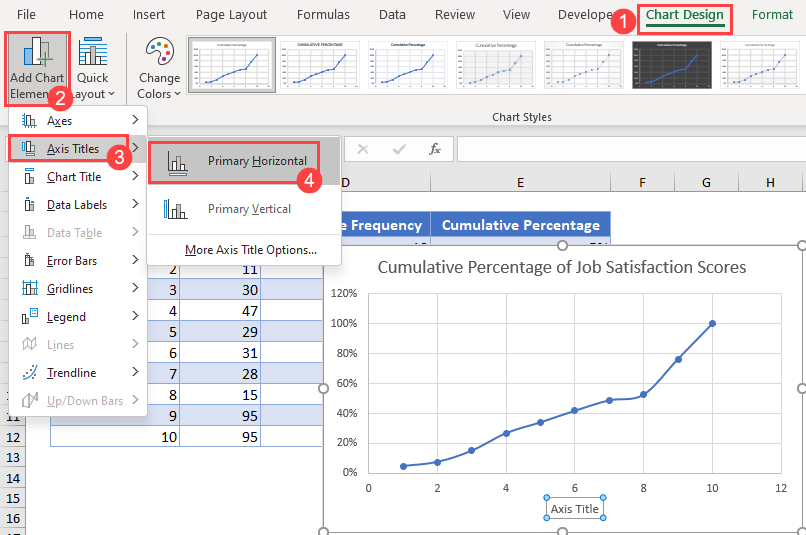Then, edit the titles as required.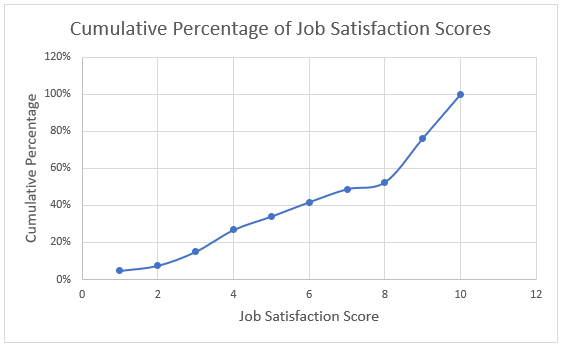# Cumulative Percentages in Google Sheets

Cumulative Percentage can be calculated in Google Sheets in a similar way as it is calculated in Excel.

First, follow the method described in the Excel section to obtain the Cumulative Percentage table.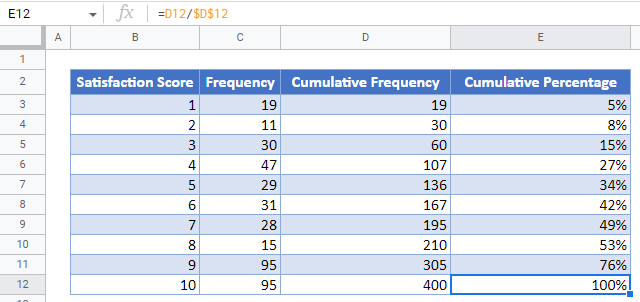To create the cumulative percentage curve (ogive) in Google Sheet, first, highlight the Satisfaction Score and the Cumulative Percentage column, and then click the Chart option from the Insert tab.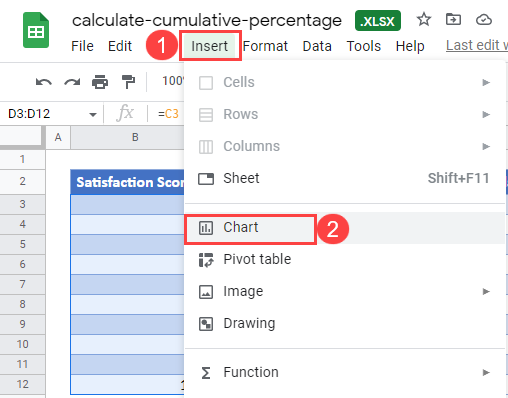On the Chart editor, change the Chart type to Smooth line chart in the Setup option.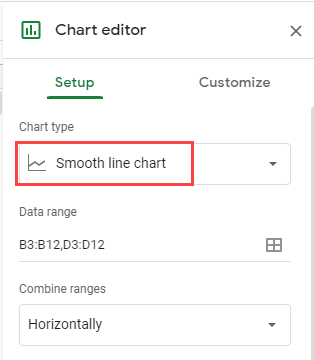The resulting graph is shown below.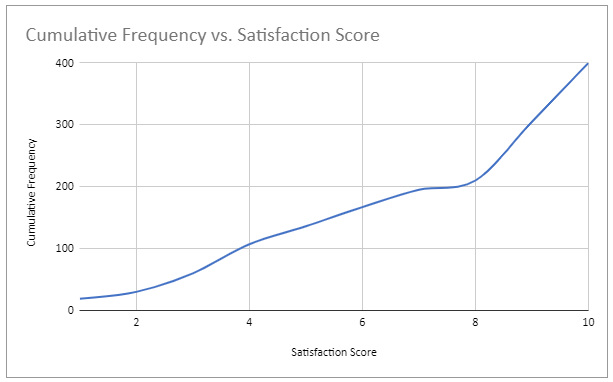To add the data point markers, click on the Customize option, then on the Series dropdown, click on Add in the Format data point section.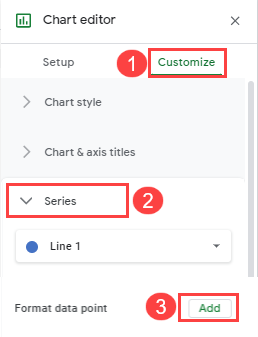On the Select data point pop-up, select a data point and click OK.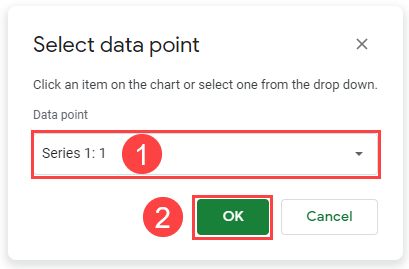Set the Color, the Point size, and the Point shape as required.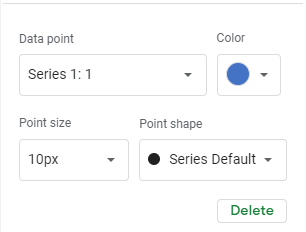Repeat the above process until you have added all the data points.

The resulting chart is shown below.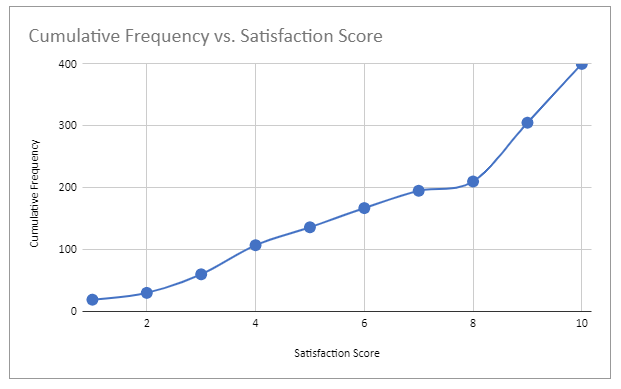An alternate way and yet, an easier hack to add the data point markers is to check the Error bars checkbox in the Format data point section and then set the Value to 0.